# From pebbles to electrons

Based on a paper by Kauffman and Noyes.

## Part I: From pebbles to combinatorics

Here's a very boring zero-player game. Take a triangular tableau of little pits, kind of like a mancala board: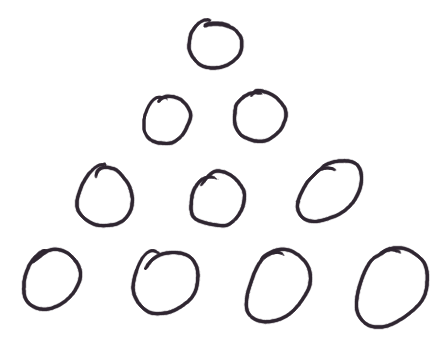Put a pebble in the top one: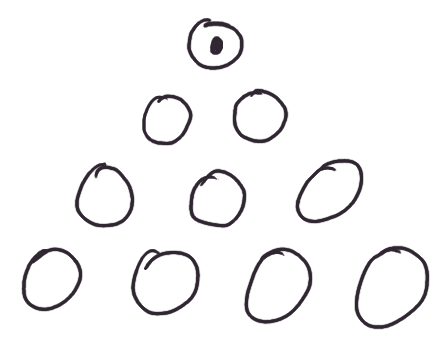And for every pebble you have, put one in each of the two pits immediately below it: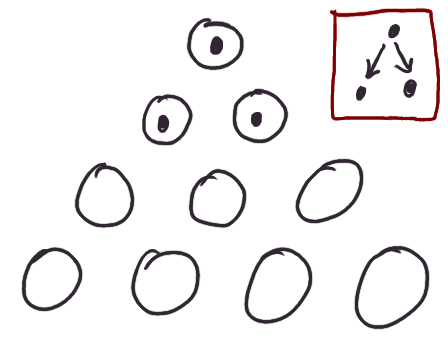Continue applying this rule all the way down: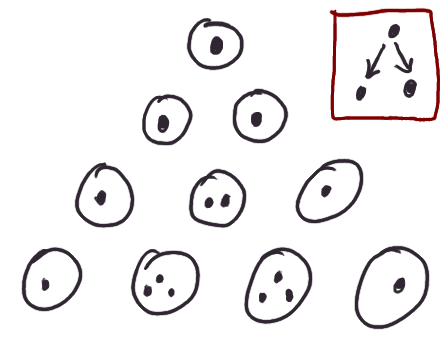Congratulations, you have just invented Pascal's Triangle, and confronted the ineffable mysteries of probability and combinatorics.

Of course, we might have decided to have different kinds of pebbles, and different rules for them. Perhaps a black pebble begets a green to the left, and a black to the right, and a green pebble begets a black pebble to the right, like so: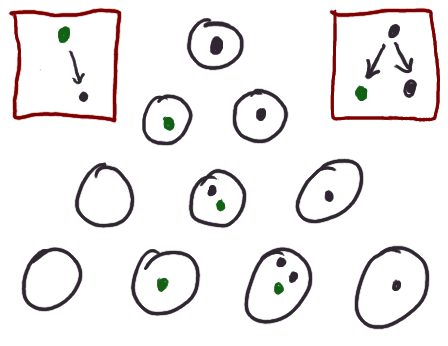And since today we are bold Mathematicians, we are comfortable employing other, more general notions of number. Below, a green pebble begets one-half of a black pebble to the right.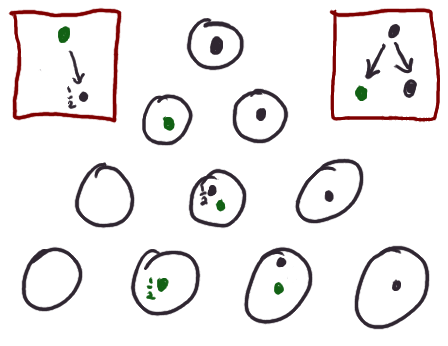Notice how the half-a-black-pebble that appeared upon the tableau works: it generated half of a green to its left, and half of a black to its right (which combined with another half-a-black that came from the green pebble in the same bin as the original half-a-black, formed a full black pebble...). This is only natural: it has half the effect of a full black pebble.

But fractions are kids' stuff. The ghost of Richard Feynman appears, and suggests we consider the following game. A black pebble begets a black pebble to its right, but also a small imaginary amount of green pebbles, iε of them, for ε a small fixed real number. A green pebble begets, to its left, a green pebble and also iε black pebbles.

It's as if black pebbles long only to travel right, and green pebbles to the left, but with a small --- and imaginary! --- probability, they change their mind, and become the other kind of particle. Ahem, pebble.

We would like to know how many pebbles we can expect to have in the distant future at a particular place in the triangular tableau.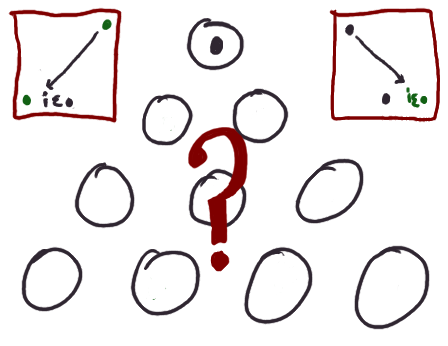Feynman's ghost, still hanging around for some reason, points out we can compute this by summing over all paths through the tableau to that place, letting each path contribute (iε)d to the sum, where d is the number of times that path changed direction.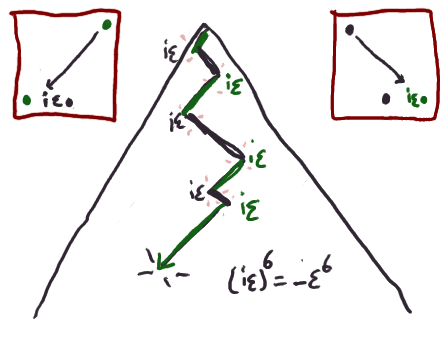We can work out an explicit formula by staring at the following diagram, and doing our best to pretend that we are not just Mathematicians, but Combinatorialists at that.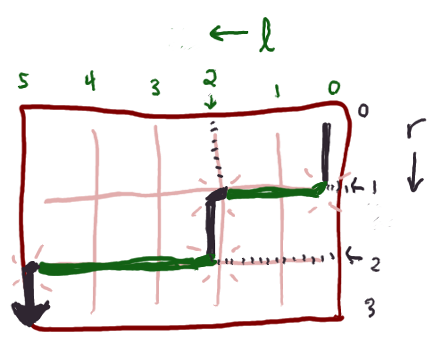Consider a path that travels a total of l hops down and to the left, and r hops down and to the right, and starts out and ends up going to the right. It's got to have an even number of kinks in it --- say it has 2k of them --- and where they are can be completely determined by a choice of k-1 places along the L-axis between 0 and l (not inclusive) where kinks happen, and a choice of k places along the R-axis between 0 and r (not inclusive) where kinks happen. The corresponding formulae for paths that start and/or end by going to the left are like so: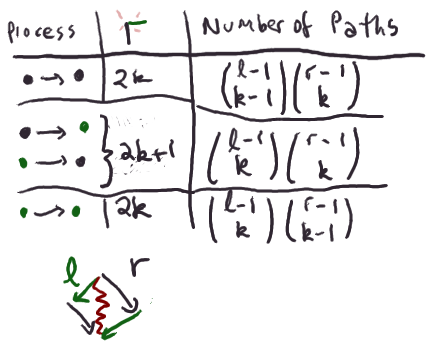And so we can return to the idea of summing over all possible paths by summing over k, and throwing in the appropriate power of iε. Here's an explicit formula for how many black and green pebbles you get at position (l,r), if you start with one black pebble: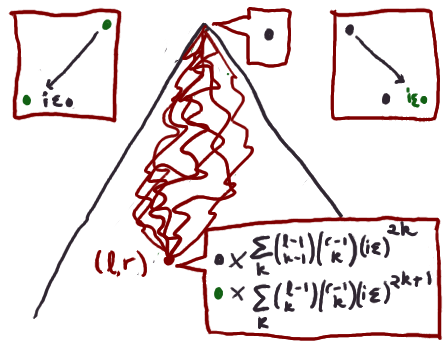## Part II: From combinatorics to electrons

What happens if r and l are really big? Then, for instance, the difference between r and r-1 isn't really a big deal, among other things. In fact, looking at the formula for r choose k, we can squinch up our eyes and make the approximation: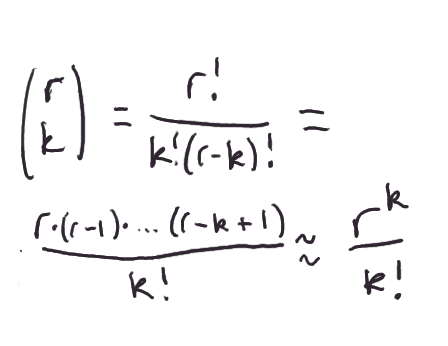Approximately, then, our explicit formula now looks like so: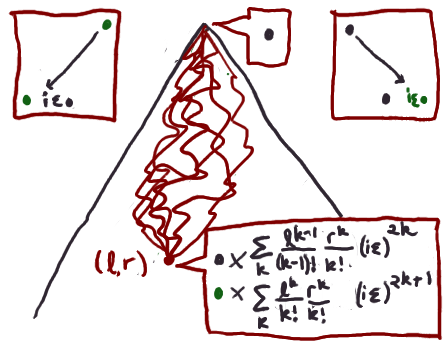Gosh, what a nice little power series in k. If we set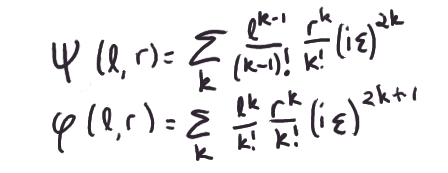then notice that we get some nice identities relating them: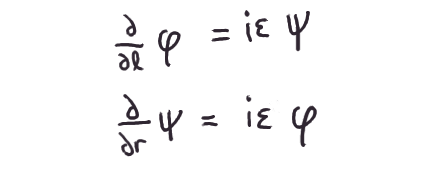Furthermore remember that taking derivatives in the r and l directions in spacetime actually correspond to differentiation operations back in a more familiar coordinate system with a spatial coordinate x and a time coordinate t: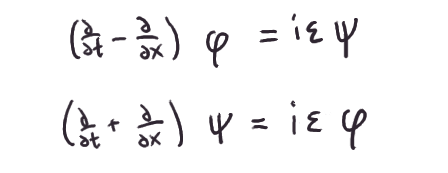What's crazy is that these two differential equations are saying that our pebbles are electrons. Sort of. At least, electrons as conceived in a mostly-broken early twentieth-century theory of special-relativistic quantum mechanics, which, despite being mostly-broken, was still of fantastic historical importance. They are the Dirac equation for a 1d universe!

I'll massage it into a more familiar form:

Go back and look at the definitions and convince yourself that we just said that if you start with a black pebble, the wavefunction ahem pebble distribution you get can be thought of as the following vector: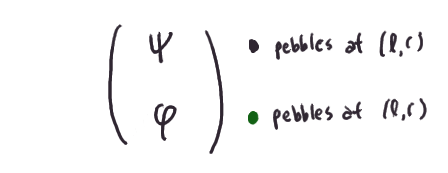Call this vector ζ. Then the above equations above are equivalent to saying: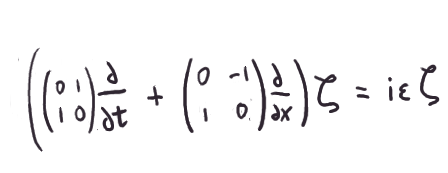All I did was express a pair of equations as a single equation on vectors, via some clever choices of matrices. By the way, everything I said up to now only dealt with the situation where you start with a black pebble, but you'd arrive at the same equation if you started with a green.

Anyhow, this is more or less the standard-looking Dirac equation. There are anti-commuting matrices latching on to the time and space derivatives on the left-hand-side. Over on the right we just have a scalar times the overall wavefunction. That is, ζ is an eigenfunction for that big operator that appears on the left-hand-side, with eigenvalue iε. Well, if it is an eigenfunction, there's no harm in hitting it with that operator twice. If we do, the anticommuting matrices do their magic and cancel out, and we're left with a version of the Klein-Gordon equation: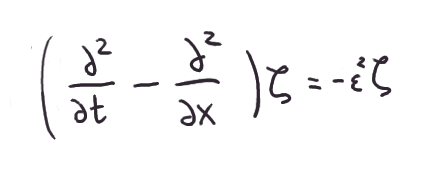Cribbing the definitions of the energy and momentum operators from wikipedia, and deciding to interpret ε as the mass of our particle, we get at last that lovely relationship among energy, momentum, and mass from special relativity: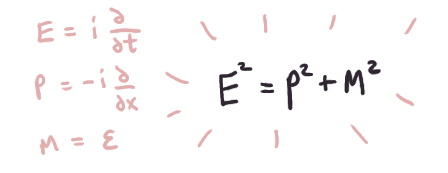And that's just one Taylor-series-expansion-of-sqrt(p^2+m^2) away from a very famous equation, indeed.

Not bad for a heap of imaginary pebbles!

Jul 12 2011